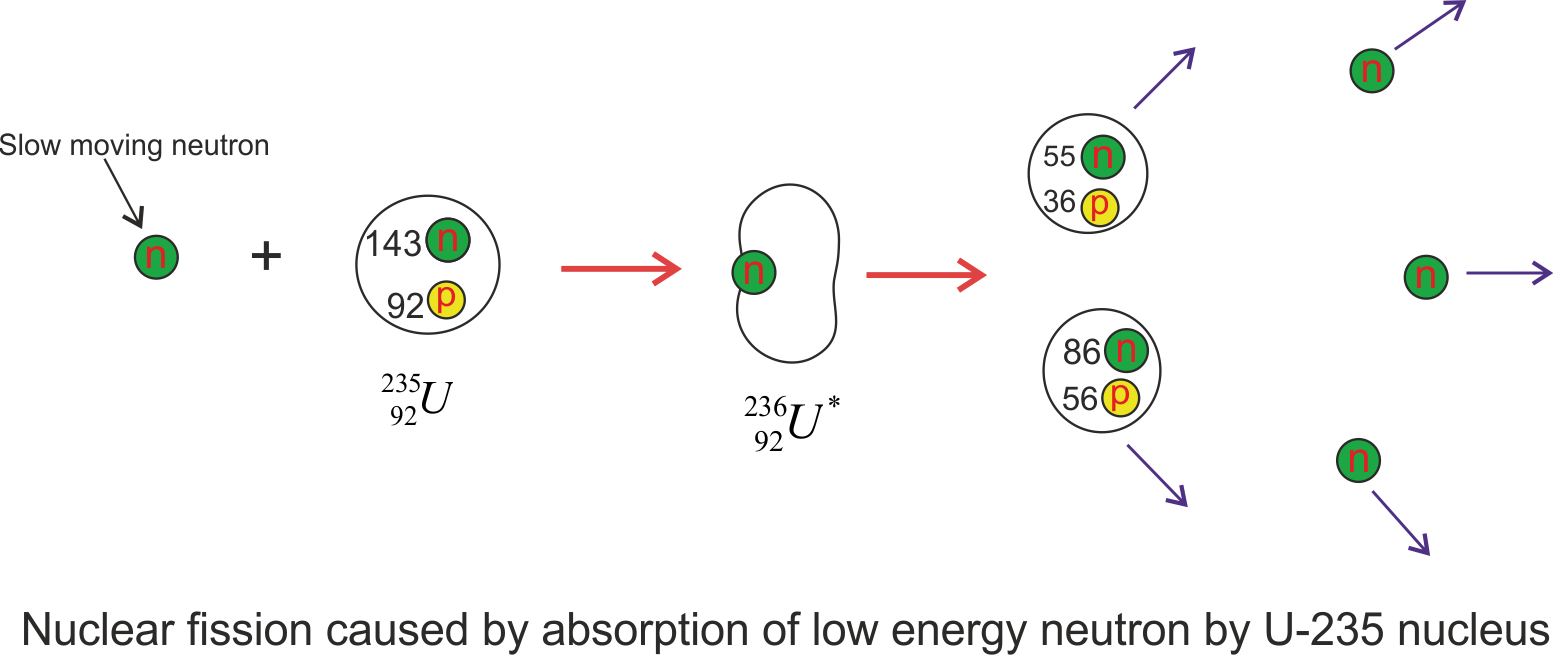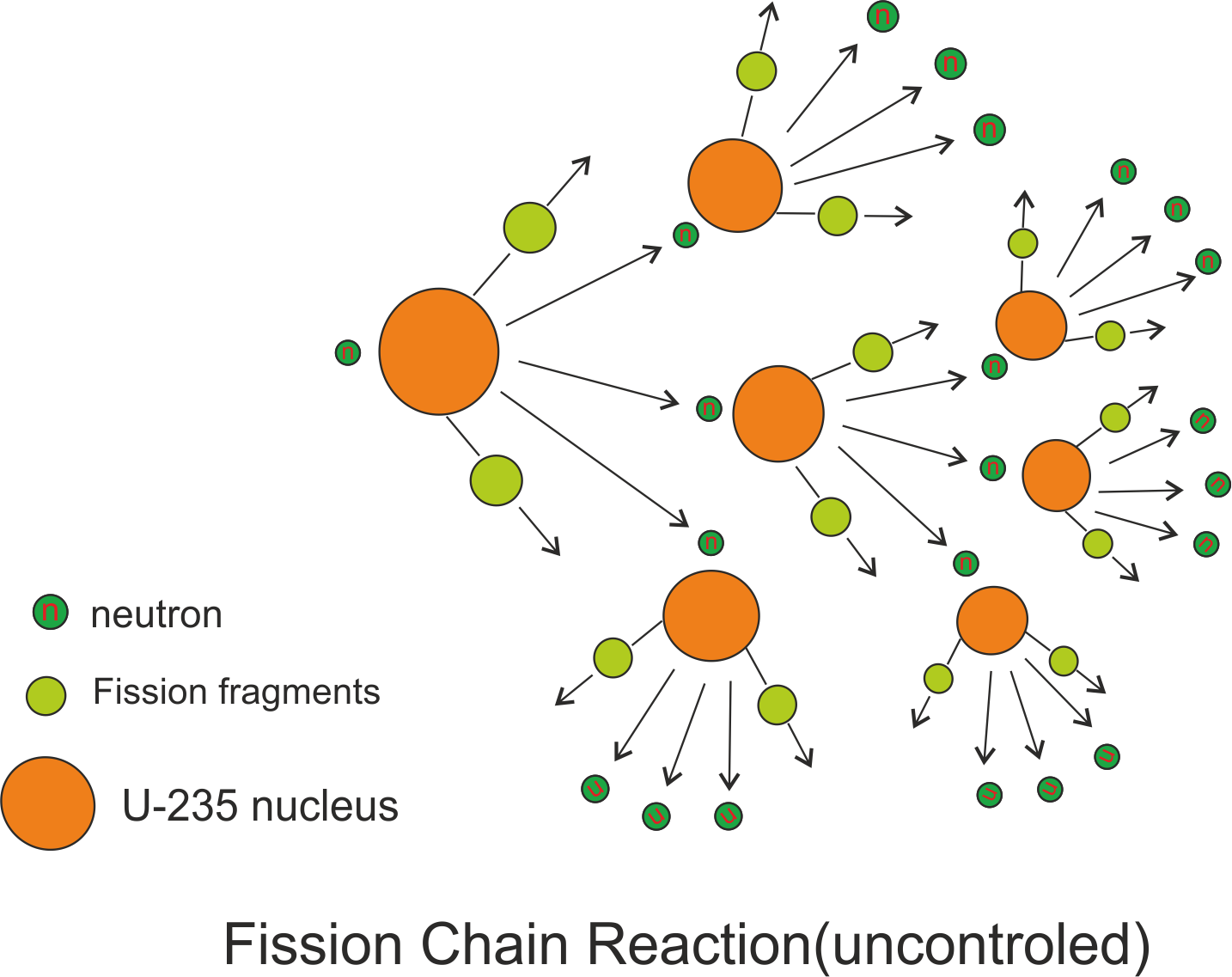Home » Physics » Nuclear Fission Basics

# Nuclear Fission Basics

[latexpage]

Nuclear fission is said to have occurred when nucleus of an atom splits into several small fragments. In nuclear physics nuclear fission either occurs as a nuclear reaction or as a radioactive decay process in which nucleus of an atom splits into smaller and lighter nuclei. The fission process often produces free neutrons and photons (in the form of gamma rays), and releases a very large amount of energy even by the energetic standards of radioactive decay.

Nuclear fission of heavy elements was discovered on December 17, 1938 by two German scientists Otto Hahn and Fritz Strassmann . They bombarded uranium (Z=92) with neutrons and they discovered Barium (Z=56) and Krypton (Z=36) were the products of their experiment. Here what happened is that the Uranium nucleus was activated by neutron bombardment and got split into two smaller nucleus. Physicists get interested in an attempt to understand this process . Along with these two smaller nucleus , three free neutrons and gamma rays were also released.  Measurements showed that about 200MeV of energy is released in each fission event.

Nuclear fission can also occur without the bombardment of external particle for example neutron and in this case the parent nucleus decays (radioactive decay) and this type of fission is called spontaneous fission and occurs in few heavy isotopes.

Now when a particle is incident on a nucleus , several things happen.  Firstly particle may scatter elastically or in-elastically which left the nucleus in the excited state which can then decay by photons or other particles. Another possibility is that the particle which was made incident on the nucleus may be absorbed by the nucleus and another particle or particles might be emitted. So this nuclear fission occurs as a nuclear reaction which is a bombardment driven process.

Here in this topic  we will learn about nuclear fission as a bombardment driven process where particle like neutrons are made incident on heavy nucleus.  Now I’ll write about fission of uranium atom by slow or low energy neutrons.

The fission of ${}^{235}U$ by slow or low energy electrons can be represented by the sequence of events

${}0^1n + {}{92}^{235}U \to {}_{92}^{236}{U^*} \to X + Y + neutrons$

where, ${}_{92}^{236}{U^*}$ is an intermediate state created during reaction and lasts only for about $10^{-12}s$ before splitting to nuclei X and Y which are known as fission fragments.  So here the nucleus absorbs the neutron, forming a highly unstable isotope that breaks up almost instantly. There are many combinations of X and Y that satisfy the requirements of conservation of energy and charge. Figure given below shows one of the ways that uranium-235 can split into two lighter nuclei.The figure given above shows the the fission of U-235 nucleus when it absorbs a slow moving neutron and results in fission fragments, barium, krypton and the released neutrons have a great deal of kinetic energy following the fission event. So  typical equation of this fission process looks like

${}0^1n + {}{92}^{235}U \to {}{92}^{236}{U^*} \to {}{56}^{141}Ba + {}_{36}^{92}Kr + 3{}_0^1n$

The process that a nucleus undergoes while fission reaction is

1. The uranium nucleus ${}^{235}U$ a slow moving and low energy thermal neutron.
2. When the nucleus captures neutron it results in the formation of an unstable isotope that is ${}_{92}^{236}{U^*}$ , and the excess energy of this nucleus causes it to undergo violent oscillations.
3. The ${}_{92}^{236}{U^*}$ nucleus becomes highly elongated, and the force of repulsion between protons in the two halves of the dumbbell-shaped nucleus tends to increase the distortion.
4. The nucleus splits into two fragments, emitting several neutrons in the process.

The energy is also released in this fission process and can also be estimated. Now a typical fission reaction releases 240 MeV of energy as stated earlier. To estimate how huge this amount is compare it to 30 eV of energy released by the explosion if a TNT molecule.  Now the combined mass of the fission fragments and the neutrons that are produced in the fission is less then the mass of the original nucleus. This small amount of mass is converted into the huge amount of energy released in fission process and is in accordance with Einstein’s mass energy equation $E=mc^{2}$. The energy of fission is mostly in the form the kinetic energy of the fission fragments and the neutrons being emitted. A small amount of energy is released in the form of Gamma radiations

## Nuclear chain reaction

A nuclear chain reaction occurs when one single nuclear reaction causes an average of one or more subsequent nuclear reactions thus leading to a self propagating series of these reactions. Now in the example of ${}^{235}U$ that undergoes fission by absorbing slow moving neutron, three neutrons are emitted . These neutrons can cause other nuclei to undergo fission with the possibility of a chain reaction as shown below in the figure.From this figure we can see that a typical fission reaction releases about three neutrons which in turn causes fission in other nuclei and results in more production of more fission fragments and more neutrons capable of triggering nuclear fission in other nuclei and so on. Thus whole chain reaction proceeds at an exponential rate  releasing tremendous amount of energy or an explosion.  However this chain reaction can be controlled in nuclear reactors and in fact this controlled chain reaction in nuclear reactors provides us electricity and is very useful.

## Nuclear Reactors

Now we know about nuclear fission and chain reaction I am going to talk about Nuclear reactors.

A nuclear reactor is a system designed to maintain self sustained chain reaction and this process was first discovered by Fermi at University of Chicago , with natural uranium as a fuel. A chain reaction can not ordinarily take place in pure natural uranium since it is mostly U-238. The neutrons released by U-235 are fast neutrons that are captured by U-238 nuclei and does not do fission. In fact slow neutrons are most likely to be captured by U-235 then by U-238. So we have to slow down the neutrons in order for fission to take place in other U-235 atom. So Enrico Fermi used graphite to slow down neutrons in the first nuclear reactor and achieved the first self-sustaining controlled release of nuclear energy.

This site uses Akismet to reduce spam. Learn how your comment data is processed.